# Sample Data: Breakdown Spots R169

Locations of electrical breakdown spots on a circular electrode of radii 169 microns in a microelectronic capacitor

## Details

Locations of electrical breakdown spots on a circular electrode of radii 169 microns in a microelectronic capacitor in a polygonal observation region bounded by the region Rectangle[{-169., -169.}, {169., 169.}] microns.

## Examples

### Basic Examples (1)

 In:=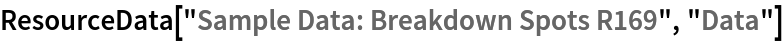Out=Summary of the spatial point data:

 In:=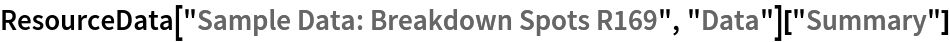Out=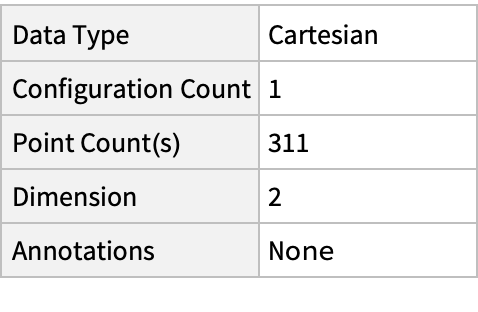### Visualizations (1)

Plot the spatial point data:

 In:=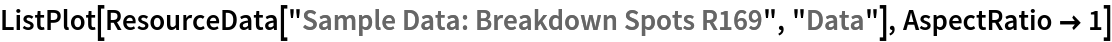Out=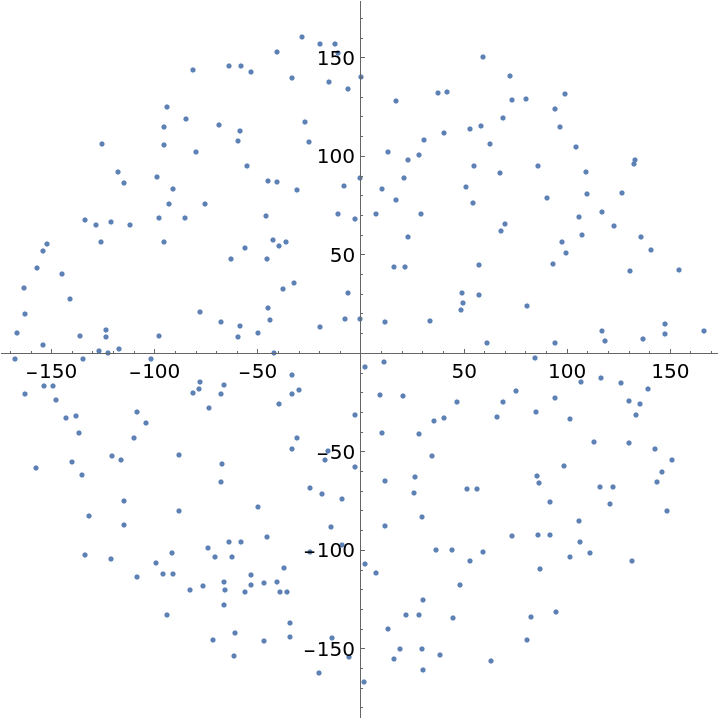### Analysis (4)

Compute probability of finding a point within given radius of an existing point - NearestNeighborG is the CDF of the nearest neighbor distribution:

 In:=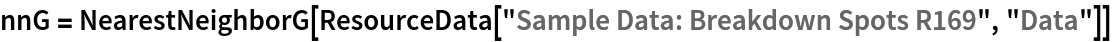Out=In:=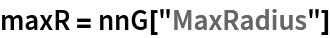Out=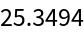In:=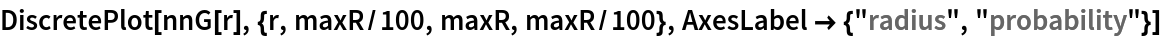Out=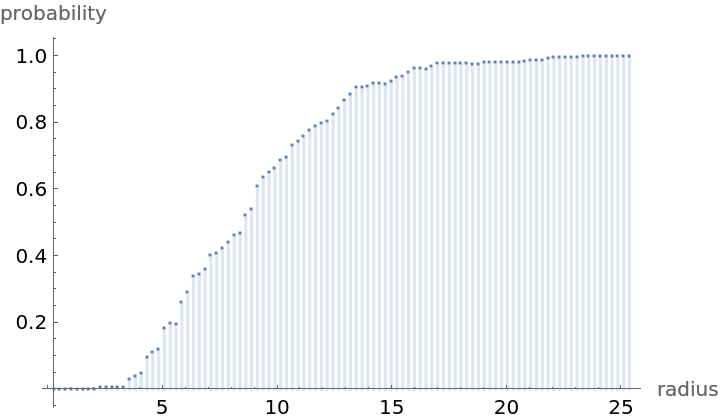Mean distance between a typical point and its nearest neighbor (for positive support distribution can be approximated via a Riemann sum of 1-CDF):

 In:=In:=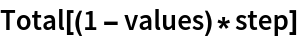Out=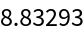Test for complete spacial randomness:

 In:=Out=Fit a Poisson point process to data:

 In:=Out=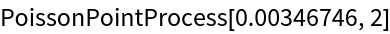Gosia Konwerska, "Sample Data: Breakdown Spots R169" from the Wolfram Data Repository (2021)